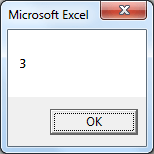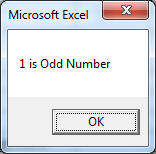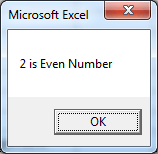# How to write a For Next Loop in Excel VBA ?

Here the term loop means, the line of written code will be repeated till the specific condition is met. In excel VBA there are different ways to run code in loops, in this article we will learn one of the method, which is For Next loop.

First we will write simple code, which will pop-up the message box for numbers 1 to 10.

1. Open new excel file and open the VBA window by pressing Alt + F11 (check for more details on How to use VBA editor and add references to Excel VBA ?)

2. Add the below lines of codes, in any of the vba module:

‘ ForLoop is the name of the macro which will appear when you press ALt + F8 to run macro
Sub ForLoop()

‘Define n variable as integer
Dim n As Integer

‘ Assign the values to variable n
For n = 1 To 10

‘MsgBox will give a pop up message with value assigned to variable n
MsgBox n

‘Next will loop back to start and add 1 to value of n, e.g. during first run of code value of n will be 1 and on execution of the Next, the value of n will become n = n + 1 i.e. n=2
Next

‘once the n value will become 10, the code will exit the execution with End Sub
End Sub

3. Run the code using Alt + F8 and choose ForLoop from the list, if you have multiple macros

4. Below is the screenshot of the third Next statement executed in the above code:Using the loop we can further write a code, which will help us to provide a message box pop-up to identify odd or even numbers.

Copy paste the below code in the VBA module:

Sub EvenOdd()
For n = 1 To 10
If n Mod 2 = 0 Then
MsgBox n & ” is Even Number”
Else
MsgBox n & ” is Odd Number”
End If
Next n
End Sub

When you will execute the above code, you will get message box pop-up saying n (value of n), if it is even number or odd number. Below is the screen shot references for both even and odd numbers.These message box pop-ups will continue till the n variable is set to 10 using For Next loop.

In above code, we have used the combination of For Next and If Else statements. e.g. If n is divisible by 2, then the number will be even, else odd. In this way, the loops can be used in different combinations in VBA code to achieve results.

Thank you for reading. Happy Learning !